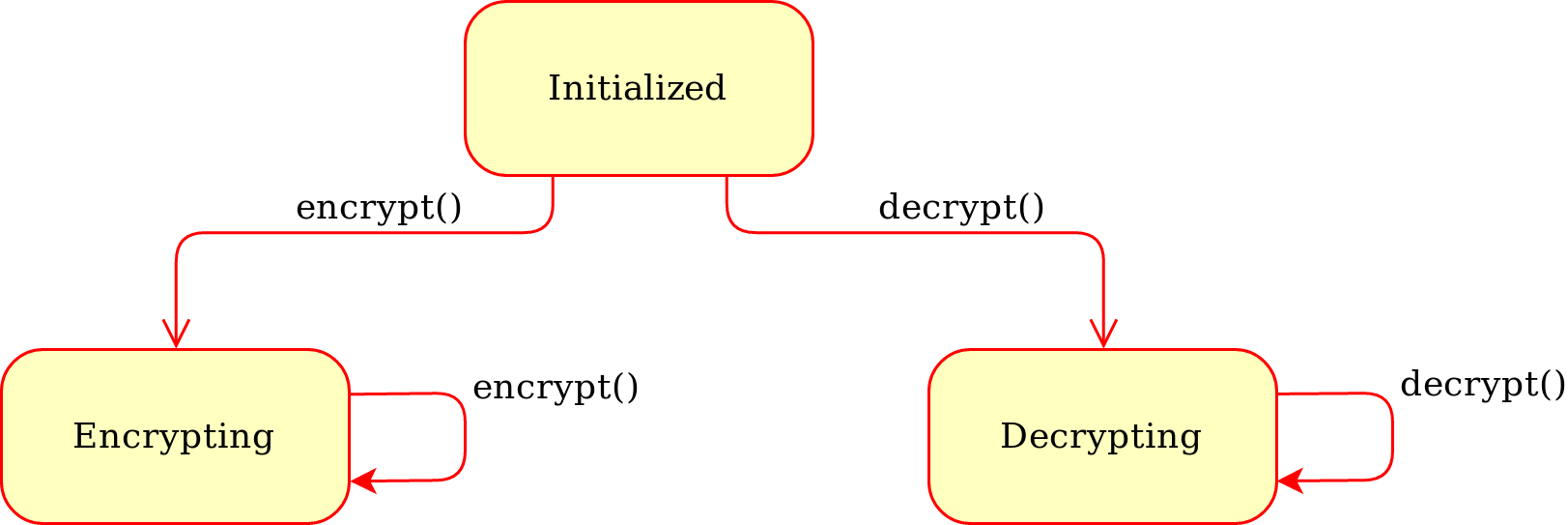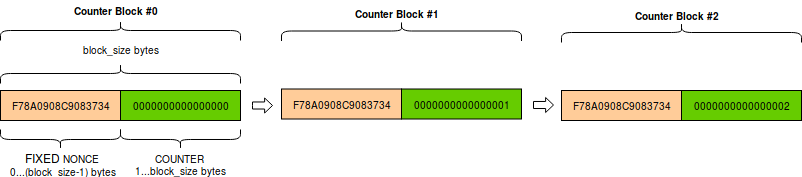# Classic modes of operation for symmetric block ciphers¶

A block cipher uses a symmetric key to encrypt data of fixed and very short length (the block size), such as 16 bytes for AES. In order to cope with data of arbitrary length, the cipher must be combined with a mode of operation.

You create a cipher object with the new() function in the relevant module under Crypto.Cipher:

1. the first parameter is always the cryptographic key (a byte string)
2. the second parameter is always the constant that selects the desired mode of operation

Constants for each mode of operation are defined at the module level for each algorithm. Their name starts with MODE_, for instance Crypto.Cipher.AES.MODE_CBC. Note that not all ciphers support all modes.

For instance:

>>> from Crypto.Cipher import AES
>>> from Crypto.Random import get_random_bytes
>>>
>>> key = get_random_bytes(16)
>>> cipher = AES.new(key, AES.MODE_CBC)
>>>
>>> # You can now use use cipher to encrypt or decrypt...


The state machine for a cipher configured with a classic mode is:Fig. 2 Generic state diagram for a cipher object

What follows is a list of classic modes of operation: they all provide confidentiality but not data integrity (unlike modern AEAD modes, which are described in another section).

## ECB mode¶

Electronic CodeBook. The most basic but also the weakest mode of operation. Each block of plaintext is encrypted independently of any other block.

Warning

The ECB mode should not be used because it is semantically insecure. For one, it exposes correlation between blocks.

The new() function at the module level under Crypto.Cipher instantiates a new ECB cipher object for the relevant base algorithm. In the following definition, <algorithm> could be AES:

Crypto.Cipher.<algorithm>.new(key, mode)

Create a new ECB object, using <algorithm> as the base block cipher.

Parameters: key (bytes) – the cryptographic key mode – the constant Crypto.Cipher..MODE_ECB an ECB cipher object

The method encrypt() (and likewise decrypt()) of an ECB cipher object expects data to have length multiple of the block size (e.g. 16 bytes for AES). You might need to use Crypto.Util.Padding to align the plaintext to the right boundary.

## CBC mode¶

Ciphertext Block Chaining, defined in NIST SP 800-38A, section 6.2. It is a mode of operation where each plaintext block gets XOR-ed with the previous ciphertext block prior to encryption.

The new() function at the module level under Crypto.Cipher instantiates a new CBC cipher object for the relevant base algorithm. In the following definition, <algorithm> could be AES:

Crypto.Cipher.<algorithm>.new(key, mode, *, iv=None)

Create a new CBC object, using <algorithm> as the base block cipher.

Parameters: key (bytes) – the cryptographic key mode – the constant Crypto.Cipher..MODE_CBC iv (bytes) – the Initialization Vector. A piece of data unpredictable to adversaries. It is as long as the block size (e.g. 16 bytes for AES). If not present, the library creates a random IV value. a CBC cipher object

The method encrypt() (and likewise decrypt()) of a CBC cipher object expects data to have length multiple of the block size (e.g. 16 bytes for AES). You might need to use Crypto.Util.Padding to align the plaintext to the right boundary.

A CBC cipher object has a read-only attribute iv, holding the Initialization Vector (bytes).

Example (encryption):

>>> import json
>>> from base64 import b64encode
>>> from Crypto.Cipher import AES
>>> from Crypto.Random import get_random_bytes
>>>
>>> data = b"secret"
>>> key = get_random_bytes(16)
>>> cipher = AES.new(key, AES.MODE_CBC)
>>> iv = b64encode(cipher.iv).decode('utf-8')
>>> ct = b64encode(ct_bytes).decode('utf-8')
>>> result = json.dumps({'iv':iv, 'ciphertext':ct})
>>> print(result)
'{"iv": "bWRHdzkzVDFJbWNBY0EwSmQ1UXFuQT09", "ciphertext": "VDdxQVo3TFFCbXIzcGpYa1lJbFFZQT09"}'


Example (decryption):

>>> import json
>>> from base64 import b64decode
>>> from Crypto.Cipher import AES
>>>
>>> # We assume that the key was securely shared beforehand
>>> try:
>>>     iv = b64decode(b64['iv'])
>>>     ct = b64decode(b64['ciphertext'])
>>>     cipher = AES.new(key, AES.MODE_CBC, iv)
>>>     print("The message was: ", pt)
>>> except ValueError, KeyError:
>>>     print("Incorrect decryption")


## CTR mode¶

CounTeR mode, defined in NIST SP 800-38A, section 6.5 and Appendix B. This mode turns the block cipher into a stream cipher. Each byte of plaintext is XOR-ed with a byte taken from a keystream: the result is the ciphertext. The keystream is generated by encrypting a sequence of counter blocks with ECB.A counter block is exactly as long as the cipher block size (e.g. 16 bytes for AES). It consist of the concatenation of two pieces:

1. a fixed nonce, set at initialization.
2. a variable counter, which gets increased by 1 for any subsequent counter block. The counter is big endian encoded.

The new() function at the module level under Crypto.Cipher instantiates a new CTR cipher object for the relevant base algorithm. In the following definition, <algorithm> could be AES:

Crypto.Cipher.<algorithm>.new(key, mode, *, nonce=None, initial_value=None, counter=None)

Create a new CTR object, using <algorithm> as the base block cipher.

Parameters: key (bytes) – the cryptographic key mode – the constant Crypto.Cipher..MODE_CTR nonce (bytes) – the value of the fixed nonce. It must be unique for the combination message/key. Its length varies from 0 to the block size minus 1. If not present, the library creates a random nonce of length equal to block size/2. initial_value (integer or bytes) – the value of the counter for the first counter block. It can be either an integer or bytes (which is the same integer, just big endian encoded). If not specified, the counter starts at 0. counter – a custom counter object created with Crypto.Util.Counter.new(). This allows the definition of a more complex counter block. a CTR cipher object

The methods encrypt() and decrypt() of a CTR cipher object accept data of any length (i.e. padding is not needed). Both raise an OverflowError exception as soon as the counter wraps around to repeat the original value.

The CTR cipher object has a read-only attribute nonce (bytes).

Example (encryption):

>>> import json
>>> from base64 import b64encode
>>> from Crypto.Cipher import AES
>>> from Crypto.Random import get_random_bytes
>>>
>>> data = b"secret"
>>> key = get_random_bytes(16)
>>> cipher = AES.new(key, AES.MODE_CTR)
>>> ct_bytes = cipher.encrypt(data)
>>> nonce = b64encode(cipher.nonce).decode('utf-8')
>>> ct = b64encode(ct_bytes).decode('utf-8')
>>> result = json.dumps({'nonce':nonce, 'ciphertext':ct})
>>> print(result)
{"nonce": "XqP8WbylRt0=", "ciphertext": "Mie5lqje"}


Example (decryption):

>>> import json
>>> from base64 import b64decode
>>> from Crypto.Cipher import AES
>>>
>>> # We assume that the key was securely shared beforehand
>>> try:
>>>     nonce = b64decode(b64['nonce'])
>>>     ct = b64decode(b64['ciphertext'])
>>>     cipher = AES.new(key, AES.MODE_CTR, nonce=nonce)
>>>     pt = cipher.decrypt(ct)
>>>     print("The message was: ", pt)
>>> except ValueError, KeyError:
>>>     print("Incorrect decryption")


## CFB mode¶

Cipher FeedBack, defined in NIST SP 800-38A, section 6.3. It is a mode of operation which turns the block cipher into a stream cipher. Each byte of plaintext is XOR-ed with a byte taken from a keystream: the result is the ciphertext.

The keystream is obtained on a per-segment basis: the plaintext is broken up in segments (from 1 byte up to the size of a block). Then, for each segment, the keystream is obtained by encrypting with the block cipher the last piece of ciphertext produced so far - possibly backfilled with the Initialization Vector, if not enough ciphertext is available yet.

The new() function at the module level under Crypto.Cipher instantiates a new CFB cipher object for the relevant base algorithm. In the following definition, <algorithm> could be AES:

Crypto.Cipher.<algorithm>.new(key, mode, *, iv=None, segment_size=8)

Create a new CFB object, using <algorithm> as the base block cipher.

Parameters: key (bytes) – the cryptographic key mode – the constant Crypto.Cipher..MODE_CFB iv (bytes) – the Initialization Vector. It must be unique for the combination message/key. It is as long as the block size (e.g. 16 bytes for AES). If not present, the library creates a random IV. segment_size (integer) – the number of bits (not bytes!) the plaintext and the ciphertext are segmented in (default if not specified: 8 bits = 1 byte). a CFB cipher object

The methods encrypt() and decrypt() of a CFB cipher object accept data of any length (i.e. padding is not needed).

The CFB cipher object has a read-only attribute iv (bytes), holding the Initialization Vector.

Example (encryption):

>>> import json
>>> from base64 import b64encode
>>> from Crypto.Cipher import AES
>>> from Crypto.Random import get_random_bytes
>>>
>>> data = b"secret"
>>> key = get_random_bytes(16)
>>> cipher = AES.new(key, AES.MODE_CFB)
>>> ct_bytes = cipher.encrypt(data)
>>> iv = b64encode(cipher.iv).decode('utf-8')
>>> ct = b64encode(ct_bytes).decode('utf-8')
>>> result = json.dumps({'iv':iv, 'ciphertext':ct})
>>> print(result)
{"iv": "VoamO23kFSOZcK1O2WiCDQ==", "ciphertext": "f8jciJ8/"}


Example (decryption):

>>> import json
>>> from base64 import b64decode
>>> from Crypto.Cipher import AES
>>>
>>> # We assume that the key was securely shared beforehand
>>> try:
>>>     iv = b64decode(b64['iv'])
>>>     ct = b64decode(b64['ciphertext'])
>>>     cipher = AES.new(key, AES.MODE_CFB, iv=iv)
>>>     pt = cipher.decrypt(ct)
>>>     print("The message was: ", pt)
>>> except ValueError, KeyError:
>>>     print("Incorrect decryption")


## OFB mode¶

Output FeedBack, defined in NIST SP 800-38A, section 6.4. It is another mode that leads to a stream cipher. Each byte of plaintext is XOR-ed with a byte taken from a keystream: the result is the ciphertext. The keystream is obtained by recursively encrypting the Initialization Vector.

The new() function at the module level under Crypto.Cipher instantiates a new OFB cipher object for the relevant base algorithm. In the following definition, <algorithm> could be AES:

Crypto.Cipher.<algorithm>.new(key, mode, *, iv=None)

Create a new OFB object, using <algorithm> as the base block cipher.

Parameters: key (bytes) – the cryptographic key mode – the constant Crypto.Cipher..MODE_OFB iv (bytes) – the Initialization Vector. It must be unique for the combination message/key. It is as long as the block size (e.g. 16 bytes for AES). If not present, the library creates a random IV. an OFB cipher object

The methods encrypt() and decrypt() of an OFB cipher object accept data of any length (i.e. padding is not needed).

The OFB cipher object has a read-only attribute iv (bytes), holding the Initialization Vector.

Example (encryption):

>>> import json
>>> from base64 import b64encode
>>> from Crypto.Cipher import AES
>>> from Crypto.Random import get_random_bytes
>>>
>>> data = b"secret"
>>> key = get_random_bytes(16)
>>> cipher = AES.new(key, AES.MODE_OFB)
>>> ct_bytes = cipher.encrypt(data)
>>> iv = b64encode(cipher.iv).decode('utf-8')
>>> ct = b64encode(ct_bytes).decode('utf-8')
>>> result = json.dumps({'iv':iv, 'ciphertext':ct})
>>> print(result)
{"iv": "NUuRJbL0UMp8+UMCk2/vQA==", "ciphertext": "XGVGc1Gw"}


Example (decryption):

>>> import json
>>> from base64 import b64decode
>>> from Crypto.Cipher import AES
>>>
>>> # We assume that the key was securely shared beforehand
>>> try:
>>>     iv = b64decode(b64['iv'])
>>>     ct = b64decode(b64['ciphertext'])
>>>     cipher = AES.new(key, AES.MODE_OFB, iv=iv)
>>>     pt = cipher.decrypt(ct)
>>>     print("The message was: ", pt)
>>> except ValueError, KeyError:
>>>     print("Incorrect decryption")


## OpenPGP mode¶

Constant: Crypto.Cipher.<cipher>.MODE_OPENPGP.

OpenPGP (defined in RFC4880). A variant of CFB, with two differences:

1. The first invocation to the encrypt() method returns the encrypted IV concatenated to the first chunk on ciphertext (as opposed to the ciphertext only). The encrypted IV is as long as the block size plus 2 more bytes.
2. When the cipher object is intended for decryption, the parameter iv to new() is the encrypted IV (and not the IV, which is still the case for encryption).

Like for CTR, an OpenPGP cipher object has a read-only attribute iv.